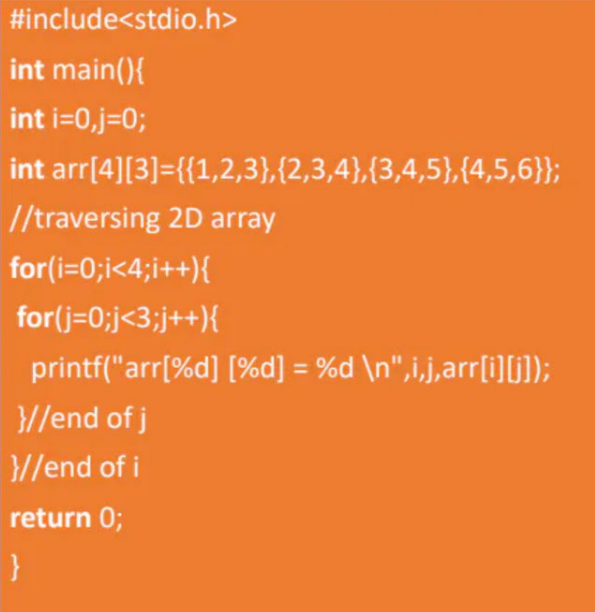### 2-D Arrays in C

• 2D Arrays(2-dimensional arrays) are represented in the form of rows and columns,also known as matrix.

• It is also known as arrays of arrays or list of arrays.

Declaration:

data_type array_name[size1][size2];

Example:

int mat=;

Where 3 is a row number and 2 is a column number.

Initialization:

int arr={{1,2,3},{4,5,6},{7,8,9},{0,1,2}};

Example of 2D Array:Output:

arr = 1

arr = 2

arr = 3

arr = 2

arr = 3

arr = 4

arr = 3

arr = 4

arr = 5

arr = 4

arr = 5

arr = 6